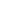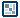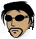## Question of the WeekChaotic Fractal Tree

by ilyas usal

The following animation is a chaotic fractal tree that is different from the previous example in the fractal tree tutorial. Keep clicking in the animation, and you will find various randomly generated branches:

[ a fractal tree...that changes with every click ]

Here, I just threw in as many Math.random() statements as I could: a random number of sub-branches, a random angle, and a random shrinking ratio. Click the scene to regenerate :)

/*** Constants ***/
max_sub_branch = 4 ;
max_sub_angle = 3*Math.PI/4 ;
max_size = 8 ;
branch_length = 50 ;

/*** Function ***/
function makeBranch ( start_x, start_y, length, angle, size ) {
if ( size > 0 ) {
this.lineStyle ( size, 0x333333, 100 ) ;
this.moveTo ( start_x, start_y ) ;
var end_x = start_x + length * Math.cos ( angle ) ;
var end_y = start_y + length * Math.sin ( angle ) ;
this.lineTo ( end_x, end_y ) ;

var sub_branch = random (max_sub_branch - 1) + 2 ;
var branch_length_dimin = .5 + Math.random()/2 ;

for ( var i=0; i < sub_branch; i ++ ) {
var newLength = length * branch_length_dimin ;
var newAngle = angle + Math.random() * max_sub_angle - max_sub_angle / 2 ;
var newSize = size - 1 ;
makeBranch ( end_x, end_y, newLength, newAngle, newSize ) ;
}
}
}

/*** Function call ***/
makeBranch ( 200, 300, branch_length, -Math.PI/2, max_size ) ;

Here, this tutorial is over! I hope you enjoyed it. And remember that fractal trees are just one aspect of what you can do with recursion. I hope that this tutorial will encourage you to discover the others.Ilyas Usal {Pictures 1 | 2}